### Home > CALC > Chapter 4 > Lesson 4.4.2 > Problem4-137

4-137.
1. Without your calculator, evaluate the following limits. Homework Help ✎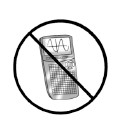1.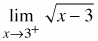2.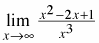3.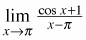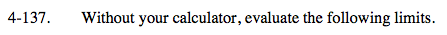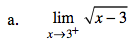Notice that this is a right-sided limit because x = 3 is an endpoint on

$y=\sqrt{x-3}.$

There is no limit from the left of the endpoint; so, consequently, this must be written as a one-sided limit.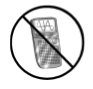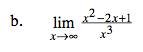This is a limit to infinity. We are looking for end-behavior. Compare the highest-power term in the numerator and the denominator.

$\lim_{x\rightarrow \infty }\frac{x^{2}}{x^{3}}=\lim_{x\rightarrow \infty }\frac{1}{x}= 0$

There is a horizontal asymptote of y = 0.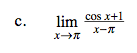This is Ana's Definition of the Derivative:

f(x) = cosx and a = π

So Ana's derivative can be evaluated as:

The limit is equal to f ' (π).